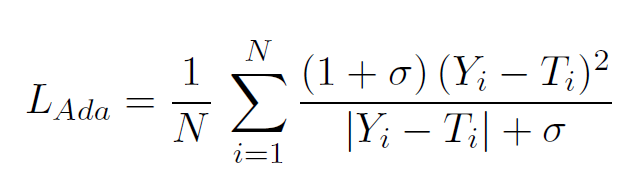I am trying to implement Adaptive Loss function, which has the formulashere my code

`````` def Adaptive_loss(preds, labels, sigma):
l2 = (1+sigma)*(torch.mean(preds - labels)**2)
l1 = sigma + torch.mean(torch.abs(preds - labels))
return l2/l1
``````

The issue I got very very worse performance compared with using MSE loss
Could anyone check if I have any mistake

You are taking `mean` in the numerator and denominator separately instead of taking the `mean` after `l2/l1`. In other words, `mean(l1/l2)` is not `mean(l1)/mean(l2)`

1 Like

Thanks a lot, your note fixed the issue

I am having difficulties implementing adaptive loss. Can you kindly share your solution function with me?